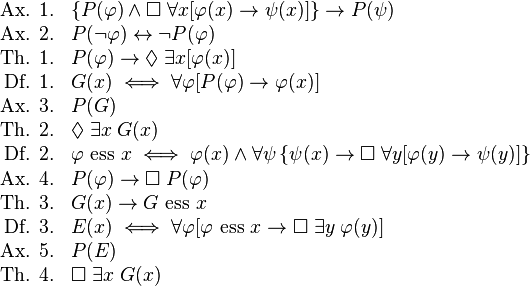## Monday, May 12, 2014

### Gödel's proof of God existence, does it work?

Kurt Friedrich Gödel (1906 – 1978) was an Austrian, and later American, logician and philosopher. One of the best mathematical minds of the 20th century. And he was brave enough to advance this ontological proof of God existence$\begin{array}{rl} \text{Ax. 1.} & \left\{P(\varphi) \wedge \Box \; \forall x[\varphi(x) \to \psi(x)]\right\} \to P(\psi) \\ \text{Ax. 2.} & P(\neg \varphi) \leftrightarrow \neg P(\varphi) \\ \text{Th. 1.} & P(\varphi) \to \Diamond \; \exists x[\varphi(x)] \\ \text{Df. 1.} & G(x) \iff \forall \varphi [P(\varphi) \to \varphi(x)] \\ \text{Ax. 3.} & P(G) \\ \text{Th. 2.} & \Diamond \; \exists x \; G(x) \\ \text{Df. 2.} & \varphi \text{ ess } x \iff \varphi(x) \wedge \forall \psi \left\{\psi(x) \to \Box \; \forall y[\varphi(y) \to \psi(y)]\right\} \\ \text{Ax. 4.} & P(\varphi) \to \Box \; P(\varphi) \\ \text{Th. 3.} & G(x) \to G \text{ ess } x \\ \text{Df. 3.} & E(x) \iff \forall \varphi[\varphi \text{ ess } x \to \Box \; \exists y \; \varphi(y)] \\ \text{Ax. 5.} & P(E) \\ \text{Th. 4.} & \Box \; \exists x \; G(x) \end{array}$ Godel's ontological proof of the existence of God (from wikipedia)
This proof uses modal logic and a very similar versions of it are explained in detail by Christopher Small here (with no previous background needed) and in this pdf.  Thanks a lot to Christopher for his good job in explaining the ontological proof.

Now, I have to say that I still need to get a deeper understanding of the proof as it is not clear to me if claiming that necessary existence is a positive property, as Gödel does (in Ax 5), is begging the question. But Gödel was a very clever guy so I need more time to think on this.

Extra notes:

St Anselm's ontological proof of God's existence is similar to Gödel's. To me, what St Anselm achieves is to clarify that, if it is possible for God to exist, then it necessarily exists.  This is basically what happens with platonic forms and mathematical theorems too. The hope is that Gödel would make a better case for the plausibility of God existence (as oppose to conceivability), and then apply Anselm's machinery to proof its existence. It is not clear to me however that Gödel have achieved the first part by means of introducing the concept of a positive property. This needs more investigation from my side.

In any case, at this stage I find Aquianas five ways far more convincing than Godel's. Aquinas doesn't go to ontological argument (and rightly criticises "Anselm's" way as a proof), instead he goes from physics, to metaphysics to God. Inductive method. Hurray for Aquinas.